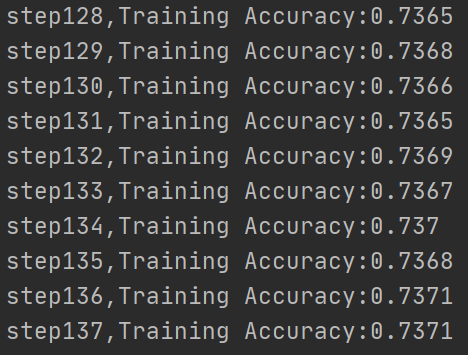Mnist手写数据集包含60000张图片作为训练数据，10000张图片作为测试集数据

from tensorflow_core.examples.tutorials.mnist import input_data
import tensorflow_core as tf
import tensorflow as tftf
#我们导入程序所需要的库函数和数据集
#注意一下，你可以好好看看这些库里面的函数
#比如这里导入input_data就是可以直接导入数据集
#或者没有数据集的话，你可以用input_data_from_github就会自动导入了
#为什么这里是tensorflow_core而不是tensorflow我也不是很清楚，但是库函数就在这里面，不然会报错
#不过你可以把两者的库函数合并，然后用tensorflow应该也没什么问题

#我们导入读取数据集，同时指定用one_hot编码的方式
batch_size = 100
#代表一次性传入神经网络进行训练的批次
batch_num = mnist_data.train.num_examples
#计算出训练的次数
#这里的train.num_examples是类里面的一个函数，我们直接用就行了，或者你打开库函数好好看看
x = tf.placeholder(tf.float32,[None,784])
#训练数据
#具体来看，我们会把训练集中的图片以batch_size批次大小，分批传入到第一个参数中去（这里默认为None）
#第二个参数是把图片转换成784的向量
y = tf.placeholder(tf.float32,[None,10])
#标签
#第二个参数是表示10个不同的类标（one_hot编码的）
weight = {
'hidden_1':tf.Variable(tf.random_normal([784,256])),
#形成一个784行256列的随机数矩阵
#这里的784：因为之前是28×28=784个像素点，也就是784个元素的向量，因此对于隐藏层来说就是784个输入
'out':tf.Variable(tf.random_normal([256,10]))
#这里的256紧接着上一个hidden_1的256，因为上一个有多少输出，下一个就有多少输入
}
biases = {
'b1':tf.Variable(tf.random_normal()),
'out':tf.Variable(tf.random_normal())
#这个偏置是一个常量，所以可以不用向量的表示方式
}
#这里只用了一层隐藏层和输出层的神经网络，所以要设置这两个层的权值w和偏置b
#隐藏层的256代表有256个神经元
#输出层10代表有10类输出
def neural_network(x):
out_layer = tf.matmul(hidden_layer_1,weight['out']) + biases['out']
return out_layer
#函数返回的是输出层的结果

result = neural_network(x)
#调用神经网络
prediction = tftf.nn.softmax(result)
#对输出的结果进行预判.softmax是预测类别
loss = tf.reduce_mean(tf.square(y-prediction))
#计算损失函数
#梯度下降法，这里的0.2表示的是学习率，对loss进行最小化
correct_pred = tf.equal(tf.argmax(y,1),tf.argmax(prediction,1))
#argmax返回函数最大的值所在的位置，在使用equal函数和正确的值进行比较，返回一个bool值，对或者错
accuracy = tf.reduce_mean(tf.cast(correct_pred,tf.float32))
#用cast函数将bool类型的预测的值转化成float类型（True转化成1，False转化成0）
#同时对所有的预测结果统计求平均值，算出最后的准确值
init = tf.global_variables_initializer()
#对程序中的所有的变量进行初始化
step_num = 400
#一共训练400次，每训练一次就会更新一次参数
#下面启动会话，程序开始启动
with tf.Session() as sess:
sess.run(init)
for step in range(step_num):
for batch in range(batch_num):
#分批处理
batch_x,batch_y = mnist_data.train.next_batch(batch_size)
#batch_x,batch_y分别表示图片的数据和图片的标签
sess.run(train_step,feed_dict={x:batch_x,y:batch_y})
#将数据填充进去
acc = sess.run(accuracy,feed_dict={x:mnist_data.test.images,y:mnist_data.test.labels})
print("step"+str(step+1)+',Training Accuracy:'+str(acc))
print('Finished!')